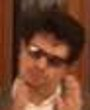Two nonlinear days in Urbino 2017. Electron. J. Diff. Eqns., Conference 25 (2018), pp. 77-85.

### Differentiability versus approximate differentiability Luigi D'Onofrio

Abstract:
One of the main tools in geometric function theory is the fact that the area formula is true for Lipschitz mapping; if f is differentiable a.e. (in the classic sense) then f can be exhausted up to a set of zero measure; the restriction of f, set by set, is Lipschitz [6, Theorem 3.18]. The aim of this survey is to clarify the regularity assumptions for a map to be differentiable a.e., and to give some auxiliary results when it is not, using the notion of approximate differentiability.

Published September 15, 2018.
Math Subject Classifications: 46E35.
Key Words: Sobolev homeomorphism; Lusin condition; approximate differentiability.

Show me the PDF file (240 K), TEX and other files for this article.Luigi D'Onofrio University of Napoli "Parthenope", Italy email: donofrio@uniparthenope.it